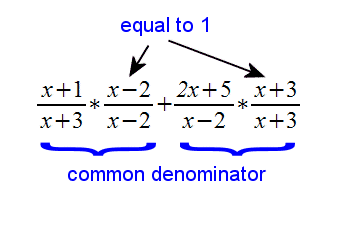# Adding and Subtracting Rational Functions

### What is a rational function again?

First of all, a rational function is pretty much just the division of two polynomial functions. For example, the following is a rational function:

$$f(x)=\frac{4x+4}{6x-9}$$

### How do we add or subtract them?

When adding or subtracting rational functions, you must find a common denominator as you might do with regular fractions. For example, to add $$\frac{1}{2}$$ and $$\frac{1}{3}$$, you might do the following:

The original fractions: $$\frac{1}{2}+\frac{1}{3}$$

with a common denominator: $$\frac{2}{6}+\frac{3}{6}$$

result: $$\frac{5}{6}$$

Now, let's apply this same strategy to the addition and subtraction of rational functions:

$$\frac{x+1}{x+3}+\frac{2x+5}{x-2} = ??$$

Step 1) Find a common denominator by multiplying the denominators. So, $$(x + 3)(x - 2)$$ becomes our common denominator in this case. Then, multiply each fraction by something equivalent to "1" (which of course doesn't change the actual value!), such as $$\frac{x+3}{x+3}$$, to get each fraction in terms of that common denominator:Here's what we have so far. Just multiply out (expand) the top and we will be ready to add the two fractions:

$$\frac{(x+1)(x-2)}{(x+3)(x-2)}+\frac{(2x+5)(x+3)}{(x+3)(x-2)}$$

$$\frac{x^2-2x+x-2}{(x+3)(x-2)}+\frac{2x^2+6x+5x+15}{(x+3)(x-2)}$$

Now add the numerators just like you would with two simple fractions:

$$\frac{3x^2+10x+13}{(x+3)(x-2)}$$

Finally we probably want to expand the denominator as well to give us the resulting rational function:

$$\frac{3x^2+10x+13}{x^2+x-6}$$

And that's our answer!

NOTE: To subtract rational functions, follow the same steps that you used to add rational functions, but just subtract the numerators instead of adding them!

For information on rational functions, try a search on Google or this lesson on rational functions.

By Mr. Feliz and Free Math Help

### Summary

Rational functions may look complicated, but we can still add or subtract by finding a common denominator.